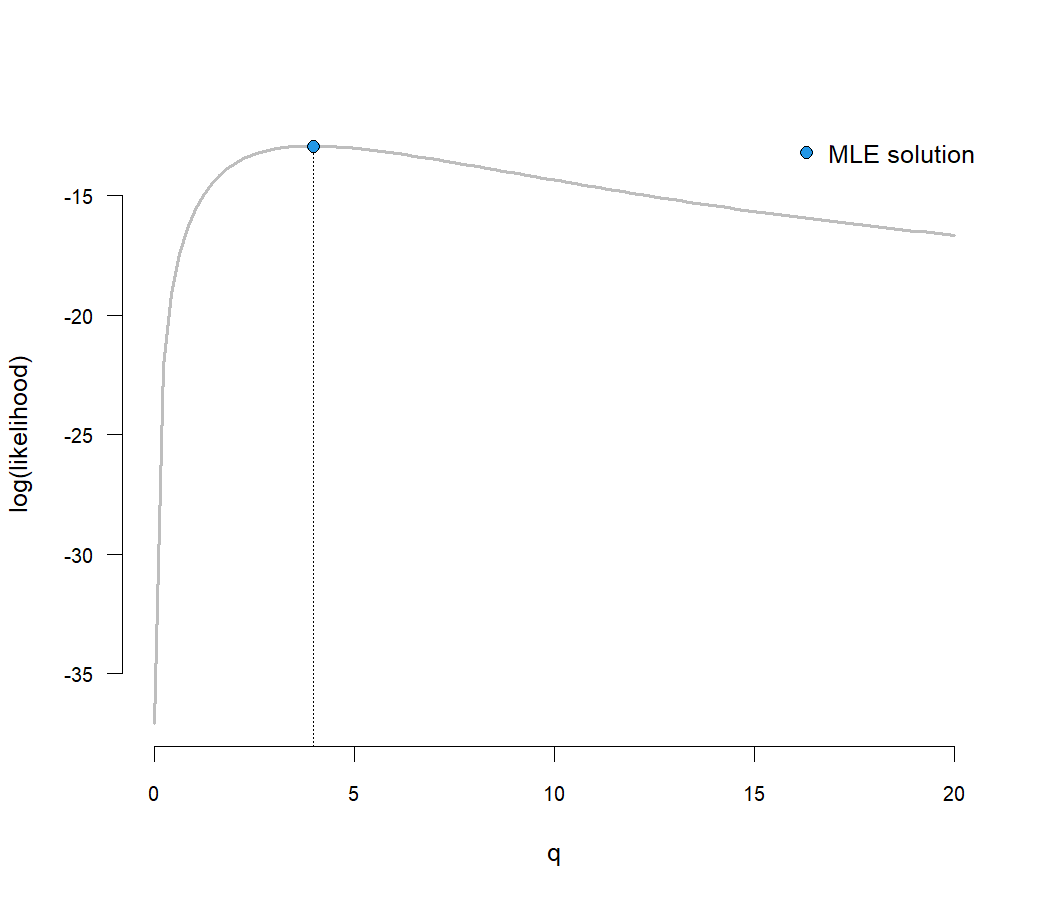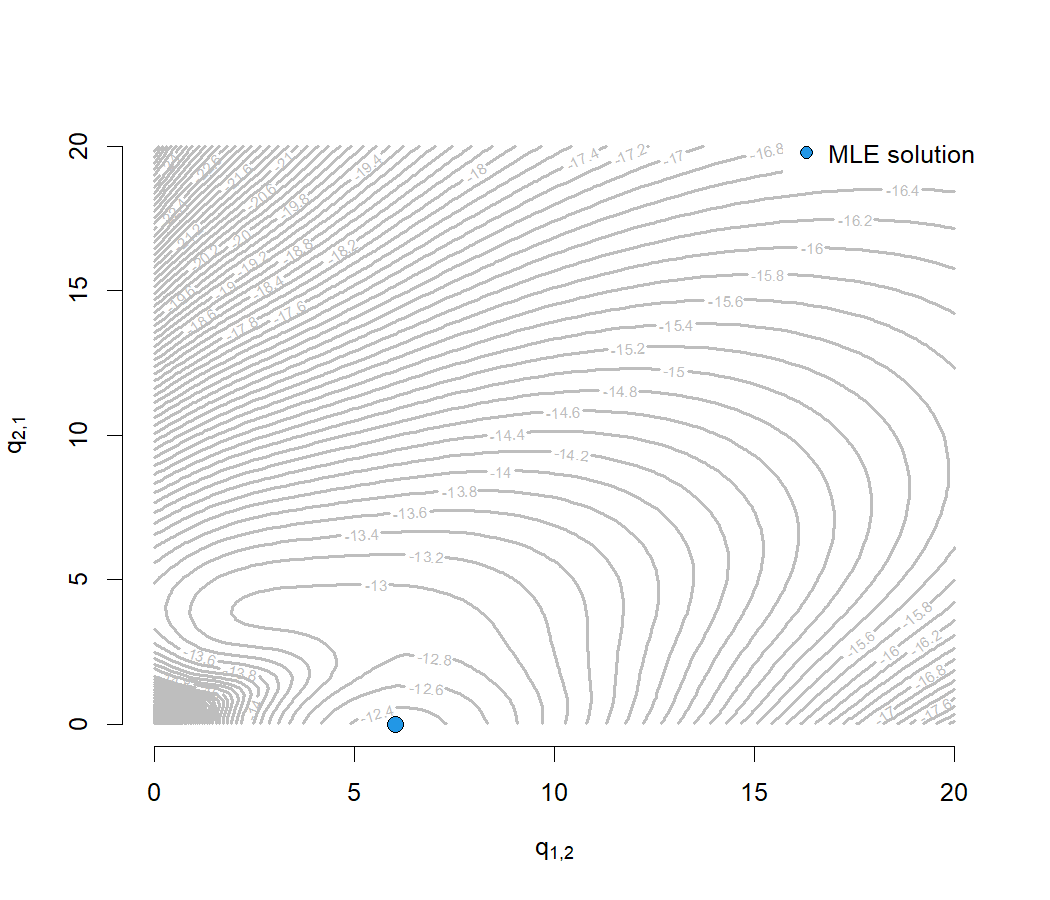## Monday, December 20, 2021

### Graphing the likelihood surface of a fitted Mk discrete character evolution model using phytools

Here's a quick tutorial on graphing the likelihood surface for an Mk model using the phytools function `fitMk`.

Essentially, our workflow is that we first fit the model using `fitMk`. It's not actually important that we find the true MLE in this step because what we end up doing is pulling the likelihood function from this model object!

Next, we use this likelihood function to compute the log-likelihood for the values of the transition rate or rates, q, over our range of interest.

Finally, we'll select an appropriate plotting method and graph our surface.

For this demo I'll use data for feeding mode (a binary trait) modified from Revell & Collar (2009).

``````library(phytools)
data(sunfish.tree)
data(sunfish.data)
## extract discrete character (feeding mode)
fmode<-setNames(sunfish.data\$feeding.mode,
rownames(sunfish.data))
``````

First, I'll compute a likelihood surface for a simple ER (equal-rates) model. This model has only one parameter, so this likelihood surface will have q on the horizontal axis, and log(L) on the vertical.

I'm going to define the range of values for q based on my pre-existing knowledge about the ML rates in this model. You should adjust to your own data accordingly.

``````## create dummy Q matrix of 1s
Q<-matrix(c(-1,1,1,-1),2,2,dimnames=list(levels(fmode),
levels(fmode)))
## get likelihood function
fit<-fitMk(sunfish.tree,fmode,pi="fitzjohn")
lik<-fit\$lik
## create vector
q<-seq(0.01,20,length.out=100)
## compute log-likelihoods
logL<-sapply(q,function(q) lik(Q*q))
## plot the surface
plot(q,logL,las=1,cex.axis=0.8,type="l",ylab="log(likelihood)",
bty="n",las=1,col="grey",lwd=2)
## add the MLE back in
lines(rep(fit\$rates,2),c(par()\$usr,logLik(fit)),lty="dotted")
points(fit\$rates,logLik(fit),pch=21,bg=palette(),cex=1.2)
legend("topright","MLE solution",pch=21,pt.bg=palette(),
pt.cex=1.2,bg="white",box.col="white")
``````Second, let's repeat this but for an ARD (all-rates-different) model.

In this case, we have a binary trait, so this just involves estimating two different parameters, and then visualizing their joint surface.

``````logL<-matrix(NA,length(q),length(q),dimnames=list(q,q))
for(i in 1:nrow(logL)) for(j in 1:ncol(logL))
logL[i,j]<-lik(matrix(c(-q[i],q[j],q[i],-q[j]),2,2))
contour(q,q,logL,nlevels=100,bty="n",col="grey",lwd=2)
title(xlab=expression(q["1,2"]),ylab=expression(q["2,1"]))
xy<-fitMk(sunfish.tree,fmode,model="ARD",pi="fitzjohn")\$rates[2:1]
points(xy,xy,cex=1.5,pch=21,bg=palette())
legend("topright","MLE solution",pch=21,pt.bg=palette(),pt.cex=1.2,
bg="white",box.col="white")
``````Cool.